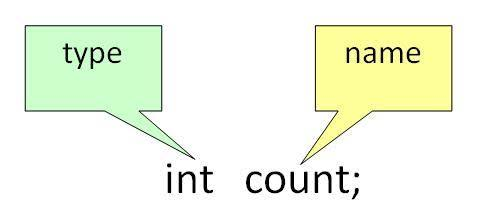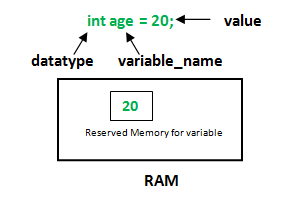# Variables in Java

• Difficulty Level : Easy
• Last Updated : 19 Apr, 2022

Variable in Java is a data container that saves the data values during Java program execution. Every variable is assigned a data type that designates the type and quantity of value it can hold. Variable is a memory location name of the data.

A variable is a name given to a memory location. It is the basic unit of storage in a program.

• The value stored in a variable can be changed during program execution.
• A variable is only a name given to a memory location, all the operations done on the variable effects that memory location.
• In Java, all the variables must be declared before use.

### How to declare variables?

We can declare variables in java as pictorially depicted below as a visual aid.From the image, it can be easily perceived that while declaring a variable, we need to take care of two things that are:

1. Datatype: Type of data that can be stored in this variable.

2. Dataname: Name was given to the variable.

In this way, a name can only be given to a memory location. It can be assigned values in two ways:

• Variable Initialization
• Assigning value by taking input

### How to initialize variables?

It can be perceived with the help of 3 components that are as follows:

• datatype: Type of data that can be stored in this variable.
• variable_name: Name given to the variable.
• value: It is the initial value stored in the variable.Illustrations:

```float simpleInterest;
// Declaring float variable```
```int time = 10, speed = 20;
// Declaring and Initializing integer variable```
```char var = 'h';
// Declaring and Initializing character variable```

### Types of Variables in Java

Now let us discuss different types of variables which are listed as follows:

1. Local Variables
2. Instance Variables
3. Static Variables

[Image_Link]https://media.geeksforgeeks.org/wp-content/uploads/2022

Let us discuss the traits of every variable been up here in detail.

1. Local Variables

A variable defined within a block or method or constructor is called a local variable.

• These variables are created when the block is entered, or the function is called and destroyed after exiting from the block or when the call returns from the function.
• The scope of these variables exists only within the block in which the variable is declared. i.e., we can access these variables only within that block.
• Initialization of the local variable is mandatory before using it in the defined scope.

## Java

 `/*package whatever //do not write package name here */``// Contributed by Shubham Jain``import` `java.io.*;` `class` `GFG {``    ``public` `static` `void` `main(String[] args)``    ``{``        ``int` `var = ``10``; ``// Declared a Local Variable``        ``// This variable is local to this main method only``        ``System.out.println(``"Local Variable: "` `+ var);``    ``}``}`
Output
`Local Variable: 10`

2. Instance Variables

Instance variables are non-static variables and are declared in a class outside any method, constructor, or block.

• As instance variables are declared in a class, these variables are created when an object of the class is created and destroyed when the object is destroyed.
• Unlike local variables, we may use access specifiers for instance variables. If we do not specify any access specifier, then the default access specifier will be used.
• Initialization of Instance Variable is not Mandatory. Its default value is 0
• Instance Variable can be accessed only by creating objects.

## Java

 `/*package whatever //do not write package name here */` `import` `java.io.*;` `class` `GFG {` `    ``public` `String geek; ``// Declared Instance Variable` `    ``public` `GFG()``    ``{ ``// Default Constructor` `        ``this``.geek = ``"Shubham Jain"``; ``// initializing Instance Variable``    ``}``//Main Method``    ``public` `static` `void` `main(String[] args)``    ``{` `        ``// Object Creation``        ``GFG name = ``new` `GFG();``        ``// Displaying O/P``        ``System.out.println(``"Geek name is: "` `+ name.geek);``    ``}``}`
Output
`Geek name is: Shubham Jain`

3. Static Variables

Static variables are also known as Class variables.

• These variables are declared similarly as instance variables. The difference is that static variables are declared using the static keyword within a class outside any method constructor or block.
• Unlike instance variables, we can only have one copy of a static variable per class irrespective of how many objects we create.
• Static variables are created at the start of program execution and destroyed automatically when execution ends.
• Initialization of Static Variable is not Mandatory. Its default value is 0
• If we access the static variable like the Instance variable (through an object), the compiler will show the warning message, which won’t halt the program. The compiler will replace the object name with the class name automatically.
• If we access the static variable without the class name, the compiler will automatically append the class name.

## Java

 `/*package whatever //do not write package name here */` `import` `java.io.*;` `class` `GFG {``  ` `  ``public` `static` `String geek = ``"Shubham Jain"``;         ``//Declared static variable``  ` `    ``public` `static` `void` `main (String[] args) {``       ` `      ``//geek variable can be accessed withod object creation``      ``//Displaying O/P``      ``//GFG.geek --> using the static variable``        ``System.out.println(``"Geek Name is : "``+GFG.geek);``    ``}``}`
Output
`Geek Name is : Shubham Jain`

### Differences between the Instance variable Vs. the Static variables

Now let us do discuss the differences between the Instance variable Vs. the Static variables

• Each object will have its copy of the instance variable, whereas We can only have one copy of a static variable per class irrespective of how many objects we create.
• Changes made in an instance variable using one object will not be reflected in other objects as each object has its own copy of the instance variable. In the case of static, changes will be reflected in other objects as static variables are common to all objects of a class.
• We can access instance variables through object references, and Static Variables can be accessed directly using the class name.

Syntax: Static and instance variables

```class GFG
{
// Static variable
static int a;

// Instance variable
int b;
} ```